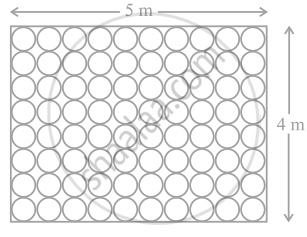# Floor of a room is of dimensions 5 m × 4 m and it is covered with circular tiles of diameters 50 cm each as shown in figure. Find the area of floor that remains uncovered with tiles. (Use π = 3.14) - Mathematics

Sum

Floor of a room is of dimensions 5 m × 4 m and it is covered with circular tiles of diameters 50 cm each as shown in figure. Find the area of floor that remains uncovered with tiles. (Use π = 3.14)#### Solution

Length of the floor = l = 5 m

Breadth of the floor = b = 4 m

∴ Area of the floor = l × b = 5 × 4 = 20 m2

Now, Diameter of each circular tile = 50 cm

∴ Radius of each circular tile = r = 25 cm = 0.25 m

Area of one circular tile = πr2

= 3.14 × (0.25)2

= 0.19625 m2

Area of such 80 tiles = 80 × 0.19625

= 15.7 m2

Area of the floor that remains uncovered with tiles =

Area of the floor – Area of all 80 circular tiles

= 20 – 15.7

= 4.3 m2

Hence, the required area of floor that remains uncovered with tiles is 4.3 m2.

Concept: Areas of Sector and Segment of a Circle
Is there an error in this question or solution?

#### APPEARS IN

NCERT Mathematics Exemplar Class 10
Chapter 11 Area Related To Circles
Exercise 11.4 | Q 11 | Page 133
Share